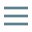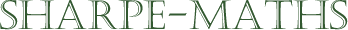Previous topic:
Multiplying brackets $\big(mx \pm a \big)\big(nx \pm b \big)$
Current topic:
Factorising expressions
Next topic:
So $4$ is a factor of $16$ and $p+1$ is a factor of $(p+1)^3$.